Select Page

# MCQ Determinant CBSE Maths 12 Science Solutions in English

MCQ Determinant CBSE Maths 12 Science Solutions in English to enable students to get Solutions in a narrative video format for the specific question.

Expert Teacher provides MCQ Determinant CBSE Maths 12 Science Solutions through Video Solutions in English language. This video solution will be useful for students to understand how to write an answer in exam in order to score more marks. This teacher uses a narrative style for a question from Determinant not only to explain the proper method of answering question, but deriving right answer too.

Please find the question below and view the Solution in a narrative video format.

Question:

Solution Video in English:

You can select video Solutions from other languages also. Please check Solutions in ( Hindi )

## Similar Questions from CBSE, 12th Science, Maths, Determinant

Question 1 : Evaluate  the determinants in :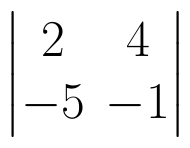. (View Answer Video)

Question 2 : Using the properties of determinants, evaluate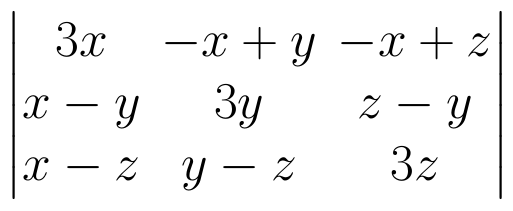. (View Answer Video)

Question 3 : If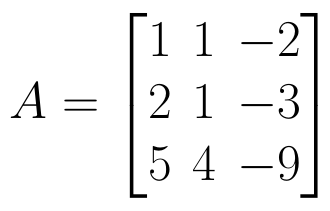find |A|. (View Answer Video)

Question 4 : Let A be a non-singular square matrix of order 3 * 3. Then | adj A | is equal to: (View Answer Video)

Question 5 : If area of a triangle is 35 sq unit with vertices (2, -6), (5, 4) and (k, 4), then k is, (View Answer Video)

### Matrices

Question 1 : Find the value of x from the equation: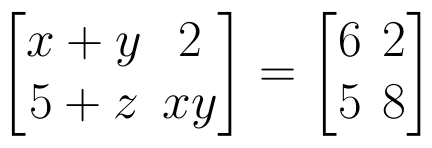. (View Answer Video)

Question 2 : If,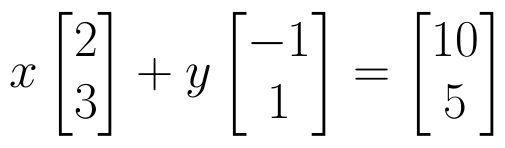find the value of y.  (View Answer Video)

Question 3 : If for any square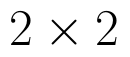matrix A,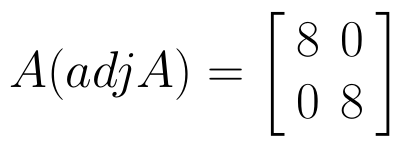then write the value of |A|. (View Answer Video)

Question 4 : Find the transpose of the matrix: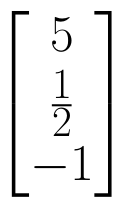. (View Answer Video)

Question 5 : Find the value of X, if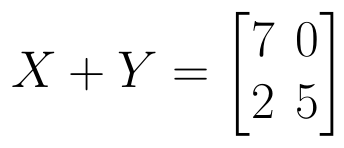and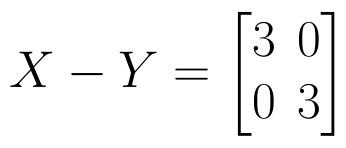. (View Answer Video)

### Relations and Functions

Question 1 : Let A = {1, 2, 3}. Then, number of relations containing (1, 2) and (1, 3) which are reflexive and symmetric but not transitive is, (View Answer Video)

Question 2 : Functions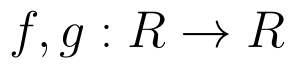are defined respectively, by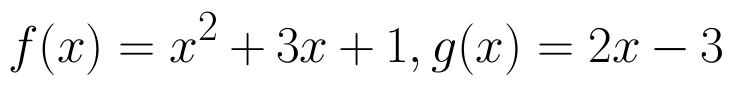, find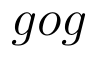(View Answer Video)

Question 3 : A functiondefined as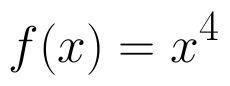is, (View Answer Video)

Question 4 :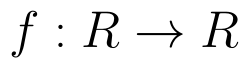be  defined as f(x) =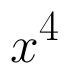(View Answer Video)

Question 5 :  * is a binary operation on Z such that: a * b = a + b + ab. The solution of (3* 4) *x = – 1 is,
Question 1 : Find the general solution of differential equation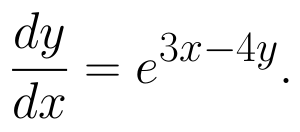(View Answer Video)
Question 3 : Find the solution of the differential equation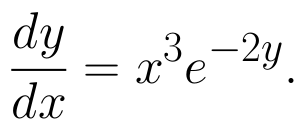(View Answer Video)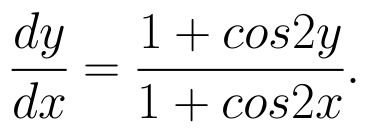(View Answer Video)